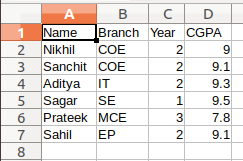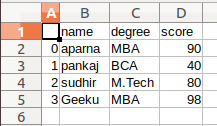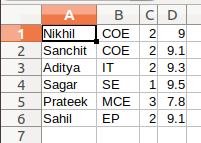# Python – Save List to CSV

• Difficulty Level : Basic
• Last Updated : 10 Jul, 2020

A CSV (Comma Separated Values) is a simple file format, used to store data in a tabular format. CSV file stores tabular data (numbers and text) in plain text. Each line of the file is a data record. Each record consists of one or more fields, separated by commas. The use of the comma as a field separator is the source of the name for this file format.

There are various methods to save lists to CSV which we will see in this article.

Method 1 : Using CSV Module

 `import` `csv ` ` `  ` `  `# field names  ` `fields ``=` `[``'Name'``, ``'Branch'``, ``'Year'``, ``'CGPA'``]  ` `   `  `# data rows of csv file  ` `rows ``=` `[ [``'Nikhil'``, ``'COE'``, ``'2'``, ``'9.0'``],  ` `         ``[``'Sanchit'``, ``'COE'``, ``'2'``, ``'9.1'``],  ` `         ``[``'Aditya'``, ``'IT'``, ``'2'``, ``'9.3'``],  ` `         ``[``'Sagar'``, ``'SE'``, ``'1'``, ``'9.5'``],  ` `         ``[``'Prateek'``, ``'MCE'``, ``'3'``, ``'7.8'``],  ` `         ``[``'Sahil'``, ``'EP'``, ``'2'``, ``'9.1'``]]  ` ` `  `with ``open``(``'GFG'``, ``'w'``) as f: ` `     `  `    ``# using csv.writer method from CSV package ` `    ``write ``=` `csv.writer(f) ` `     `  `    ``write.writerow(fields) ` `    ``write.writerows(rows) `

Output:Method 2 : Using Pandas

 `# importing pandas as pd   ` `import` `pandas as pd   ` ` `  `    `  `# list of name, degree, score  ` `nme ``=` `[``"aparna"``, ``"pankaj"``, ``"sudhir"``, ``"Geeku"``]  ` `deg ``=` `[``"MBA"``, ``"BCA"``, ``"M.Tech"``, ``"MBA"``]  ` `scr ``=` `[``90``, ``40``, ``80``, ``98``]  ` `    `  `# dictionary of lists   ` `dict` `=` `{``'name'``: nme, ``'degree'``: deg, ``'score'``: scr}   ` `      `  `df ``=` `pd.DataFrame(``dict``)  ` `   `  `# saving the dataframe  ` `df.to_csv(``'GFG.csv'``)  `

Output:Method 3 : Using Numpy

 `import` `numpy as np ` ` `  ` `  `# data rows of csv file  ` `rows ``=` `[ [``'Nikhil'``, ``'COE'``, ``'2'``, ``'9.0'``],  ` `         ``[``'Sanchit'``, ``'COE'``, ``'2'``, ``'9.1'``],  ` `         ``[``'Aditya'``, ``'IT'``, ``'2'``, ``'9.3'``],  ` `         ``[``'Sagar'``, ``'SE'``, ``'1'``, ``'9.5'``],  ` `         ``[``'Prateek'``, ``'MCE'``, ``'3'``, ``'7.8'``],  ` `         ``[``'Sahil'``, ``'EP'``, ``'2'``, ``'9.1'``]]  ` ` `  `# using the savetxt  ` `# from the numpy module ` `np.savetxt(``"GFG.csv"``,  ` `           ``rows, ` `           ``delimiter ``=``", "``,  ` `           ``fmt ``=``'% s'``) `

Output:My Personal Notes arrow_drop_up
Recommended Articles
Page :